Publicité

# cscript_controller.pdf

VcTrn1
30 Mar 2023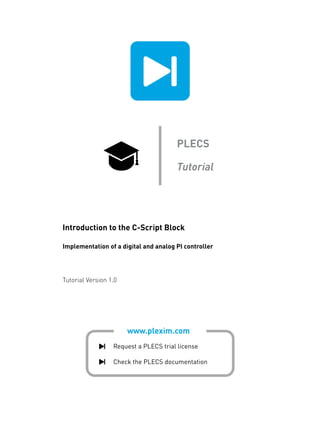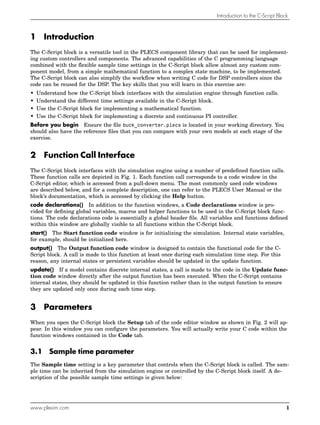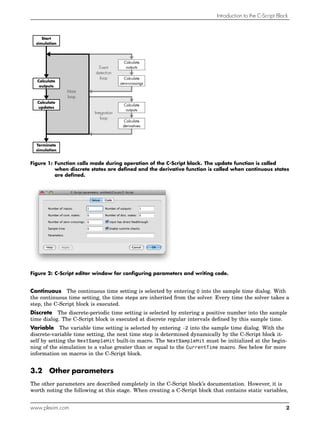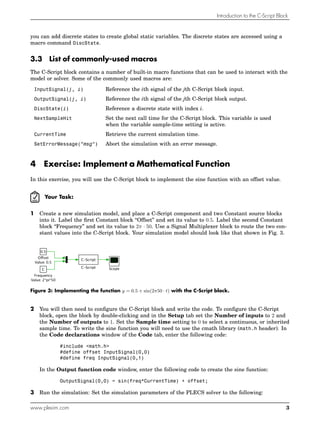Publicité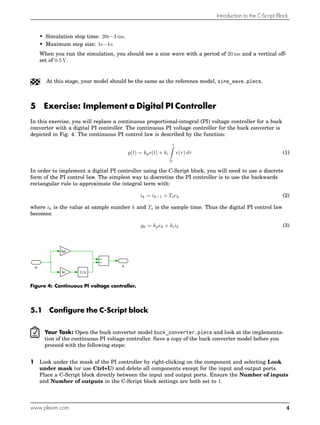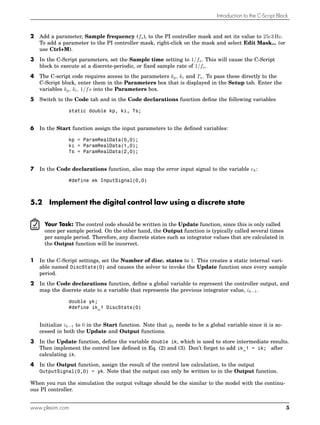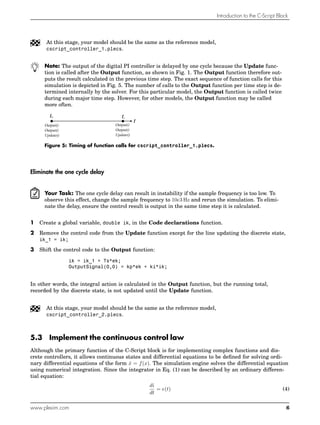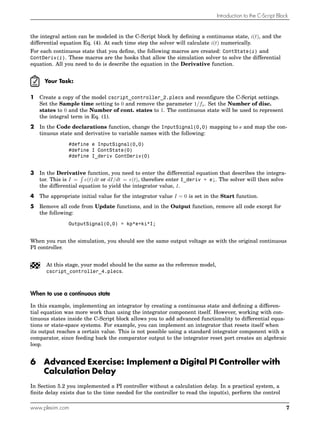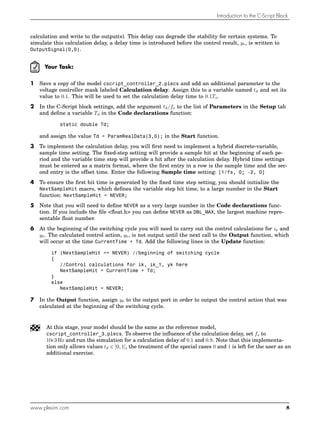Publicité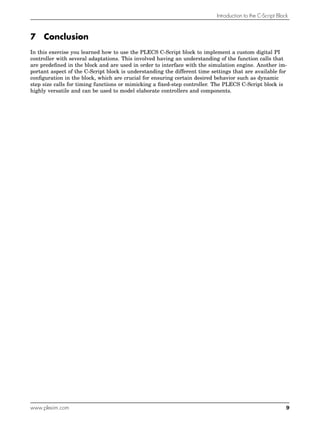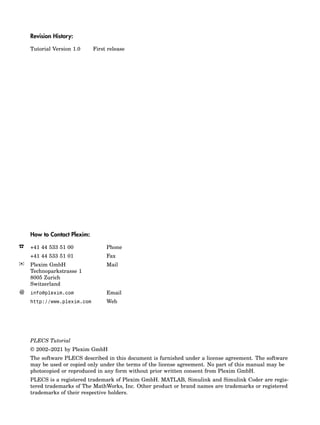Prochain SlideShare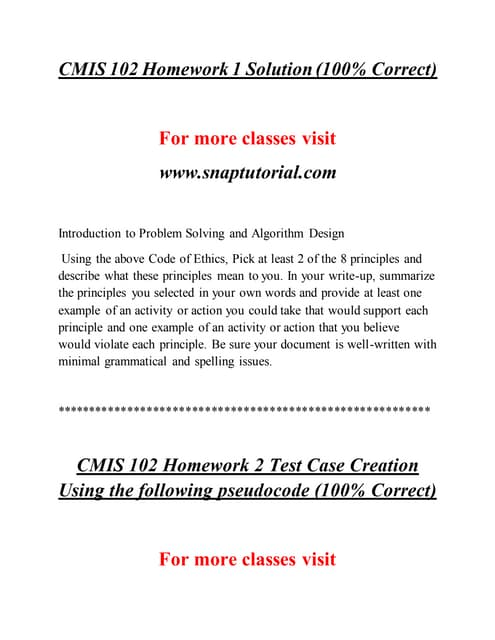Cmis 102 Success Begins / snaptutorial.com
Chargement dans ... 3
1 sur 11
Publicité

### cscript_controller.pdf

1. www.plexim.com Request a PLECS trial license Check the PLECS documentation PLECS Tutorial Introduction to the C-Script Block Implementation of a digital and analog PI controller Tutorial Version 1.0
2. Introduction to the C-Script Block 1 Introduction The C-Script block is a versatile tool in the PLECS component library that can be used for implement- ing custom controllers and components. The advanced capabilities of the C programming language combined with the flexible sample time settings in the C-Script block allow almost any custom com- ponent model, from a simple mathematical function to a complex state machine, to be implemented. The C-Script block can also simplify the workflow when writing C code for DSP controllers since the code can be reused for the DSP. The key skills that you will learn in this exercise are: • Understand how the C-Script block interfaces with the simulation engine through function calls. • Understand the different time settings available in the C-Script block. • Use the C-Script block for implementing a mathematical function. • Use the C-Script block for implementing a discrete and continuous PI controller. Before you begin Ensure the file buck_converter.plecs is located in your working directory. You should also have the reference files that you can compare with your own models at each stage of the exercise. 2 Function Call Interface The C-Script block interfaces with the simulation engine using a number of predefined function calls. These function calls are depicted in Fig. 1. Each function call corresponds to a code window in the C-Script editor, which is accessed from a pull-down menu. The most commonly used code windows are described below, and for a complete description, one can refer to the PLECS User Manual or the block’s documentation, which is accessed by clicking the Help button. code declarations() In addition to the function windows, a Code declarations window is pro- vided for defining global variables, macros and helper functions to be used in the C-Script block func- tions. The code declarations code is essentially a global header file. All variables and functions defined within this window are globally visible to all functions within the C-Script block. start() The Start function code window is for initializing the simulation. Internal state variables, for example, should be initialized here. output() The Output function code window is designed to contain the functional code for the C- Script block. A call is made to this function at least once during each simulation time step. For this reason, any internal states or persistent variables should be updated in the update function. update() If a model contains discrete internal states, a call is made to the code in the Update func- tion code window directly after the output function has been executed. When the C-Script contains internal states, they should be updated in this function rather than in the output function to ensure they are updated only once during each time step. 3 Parameters When you open the C-Script block the Setup tab of the code editor window as shown in Fig. 2 will ap- pear. In this window you can configure the parameters. You will actually write your C code within the function windows contained in the Code tab. 3.1 Sample time parameter The Sample time setting is a key parameter that controls when the C-Script block is called. The sam- ple time can be inherited from the simulation engine or controlled by the C-Script block itself. A de- scription of the possible sample time settings is given below: www.plexim.com 1
3. Introduction to the C-Script Block Calculate outputs Calculate updates Calculate outputs Calculate outputs Start simulation Terminate simulation Main loop Event detection loop Integration loop Calculate derivatives Calculate zero-crossings Figure 1: Function calls made during operation of the C-Script block. The update function is called when discrete states are defined and the derivative function is called when continuous states are defined. Figure 2: C-Script editor window for configuring parameters and writing code. Continuous The continuous time setting is selected by entering 0 into the sample time dialog. With the continuous time setting, the time steps are inherited from the solver. Every time the solver takes a step, the C-Script block is executed. Discrete The discrete-periodic time setting is selected by entering a positive number into the sample time dialog. The C-Script block is executed at discrete regular intervals defined by this sample time. Variable The variable time setting is selected by entering -2 into the sample time dialog. With the discrete-variable time setting, the next time step is determined dynamically by the C-Script block it- self by setting the NextSampleHit built-in macro. The NextSampleHit must be initialized at the begin- ning of the simulation to a value greater than or equal to the CurrentTime macro. See below for more information on macros in the C-Script block. 3.2 Other parameters The other parameters are described completely in the C-Script block’s documentation. However, it is worth noting the following at this stage. When creating a C-Script block that contains static variables, www.plexim.com 2
4. Introduction to the C-Script Block you can add discrete states to create global static variables. The discrete states are accessed using a macro command DiscState. 3.3 List of commonly-used macros The C-Script block contains a number of built-in macro functions that can be used to interact with the model or solver. Some of the commonly used macros are: InputSignal(j, i) Reference the ith signal of the jth C-Script block input. OutputSignal(j, i) Reference the ith signal of the jth C-Script block output. DiscState(i) Reference a discrete state with index i. NextSampleHit Set the next call time for the C-Script block. This variable is used when the variable sample-time setting is active. CurrentTime Retrieve the current simulation time. SetErrorMessage(”msg”) Abort the simulation with an error message. 4 Exercise: Implement a Mathematical Function In this exercise, you will use the C-Script block to implement the sine function with an offset value. Your Task: 1 Create a new simulation model, and place a C-Script component and two Constant source blocks into it. Label the first Constant block “Offset” and set its value to 0.5. Label the second Constant block “Frequency” and set its value to 2π · 50. Use a Signal Multiplexer block to route the two con- stant values into the C-Script block. Your simulation model should look like that shown in Fig. 3. Frequency Value: 2*pi*50 C Value: 0.5 Oﬀset 0.5 C-Script C-Script Scope Figure 3: Implementing the function y = 0.5 + sin(2π50 · t) with the C-Script block. 2 You will then need to configure the C-Script block and write the code. To configure the C-Script block, open the block by double-clicking and in the Setup tab set the Number of inputs to 2 and the Number of outputs to 1. Set the Sample time setting to 0 to select a continuous, or inherited sample time. To write the sine function you will need to use the cmath library (math.h header). In the Code declarations window of the Code tab, enter the following code: #include <math.h> #define offset InputSignal(0,0) #define freq InputSignal(0,1) In the Output function code window, enter the following code to create the sine function: OutputSignal(0,0) = sin(freq*CurrentTime) + offset; 3 Run the simulation: Set the simulation parameters of the PLECS solver to the following: www.plexim.com 3
5. Introduction to the C-Script Block • Simulation stop time: 20e−3 ms. • Maximum step size: 1e−4 s When you run the simulation, you should see a sine wave with a period of 20 ms and a vertical off- set of 0.5 V. At this stage, your model should be the same as the reference model, sine_wave.plecs. 5 Exercise: Implement a Digital PI Controller In this exercise, you will replace a continuous proportional-integral (PI) voltage controller for a buck converter with a digital PI controller. The continuous PI voltage controller for the buck converter is depicted in Fig. 4. The continuous PI control law is described by the function: y(t) = kpe(t) + ki t ˆ 0 e(τ) dτ (1) In order to implement a digital PI controller using the C-Script block, you will need to use a discrete form of the PI control law. The simplest way to discretize the PI controller is to use the backwards rectangular rule to approximate the integral term with: ik = ik−1 + Tsek (2) where ik is the value at sample number k and Ts is the sample time. Thus the digital PI control law becomes: yk = kpek + kiik (3) ki kp 1/s y e + + Figure 4: Continuous PI voltage controller. 5.1 Configure the C-Script block Your Task: Open the buck converter model buck_converter.plecs and look at the implementa- tion of the continuous PI voltage controller. Save a copy of the buck converter model before you proceed with the following steps: 1 Look under the mask of the PI controller by right-clicking on the component and selecting Look under mask (or use Ctrl+U) and delete all components except for the input and output ports. Place a C-Script block directly between the input and output ports. Ensure the Number of inputs and Number of outputs in the C-Script block settings are both set to 1. www.plexim.com 4